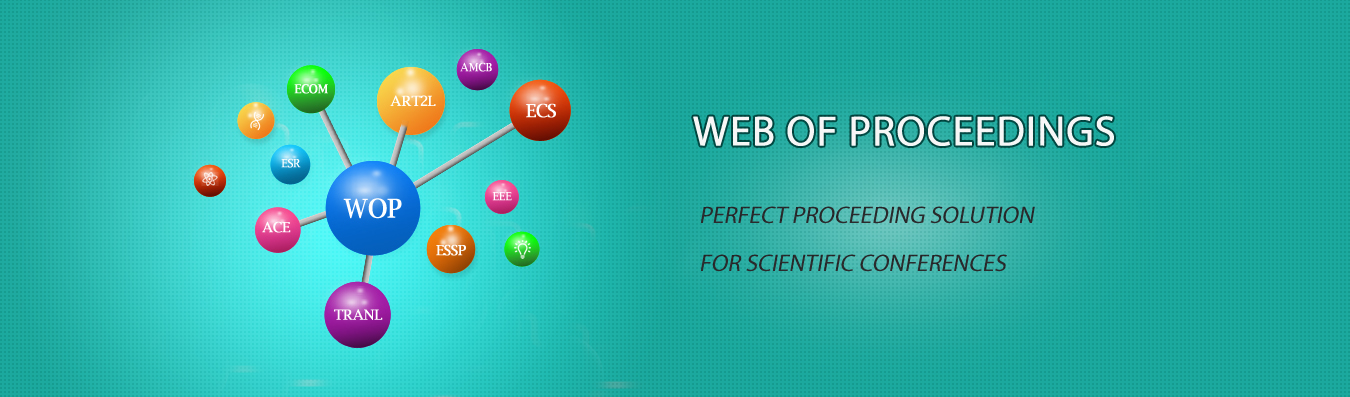The best way to conference proceedings by Francis Academic Press### Creation and Optimization of Laser Marking Hatch Contour Generation Algorithm

DOI: 10.25236/icited.2021.098

#### Author(s)

Zhaohui Wang, Yalong Bai, Xiangyu Luo

Zhaohui Wang

#### Abstract

Laser marking has been increasingly applied to industrial processing. In this paper, by studying Zigzag Parallel and Contour Parallel Hatch, we established mathematical models, designed and optimized algorithms. We first draw the fitted edge line, then find the intersection of the horizontal line and edge line. We propose an algorithm to solve this problem, named Bottom to Top Crossing Line Algorithm, we draw edge line and hatch line，and choose hatching paths based on the number of intersections. By using two given parameter groups, we establish the Space Cost Function to calculate the total length, and the Time Cost Function to calculate the average running time.And we use a similar strategy, the difference lies in the processing of the picture common nested part. The increase in the number of intersection points of Edge line and Hatch Line make the algorithm more complicated. Therefore, we divide it into several areas to hatch, and also calculate the two sets of data. Then propose two improved algorithm models. 1)An improved linear edge path optimization model based on curvature value, which is used to improve accuracy and efficiency of zigzag parallel hatching. 2) An improved algorithm model based on particle swarm optimization, which is used to shorten the time of searching seed points when laser marking multi-layer contour pattern, especially when the diameter of the marking spot is very small. The random particle swarm in the specified boundary moves continuously and calculates the distance from the contour boundary. We calculate time cost, space cost and improve rate of the two after improving our model. Also, we found when IHdistance∈[0.07,0.1](for contour, it’s [0.06,0.1]), improve rate is little, elapsed time and total lengths have a less change, but when IHdistance≤0.06(contour’s 0.05), the two parameters change a lot.

#### Keywords

Particle swarm optimization; Edge path optimization; Space Cost Function; Time Cost Function; Bottom to Top Crossing Line Algorithm